Examples

Chapter 1 Class 9 Number Systems
Serial order wise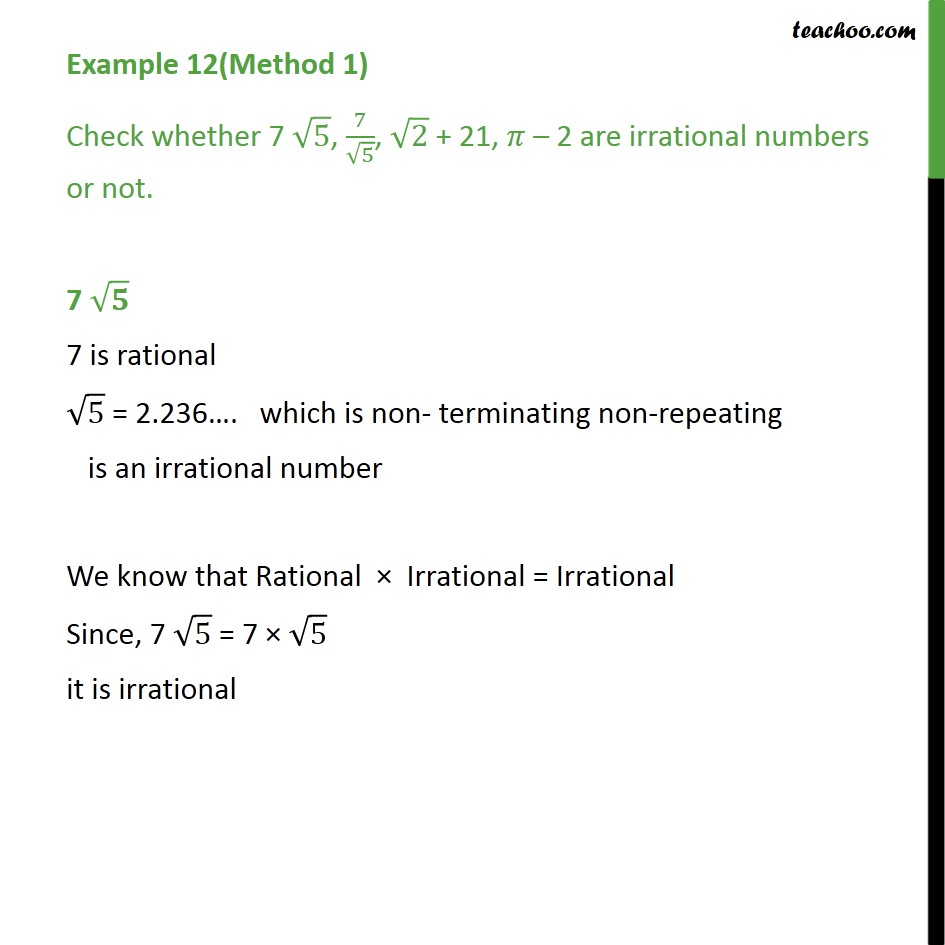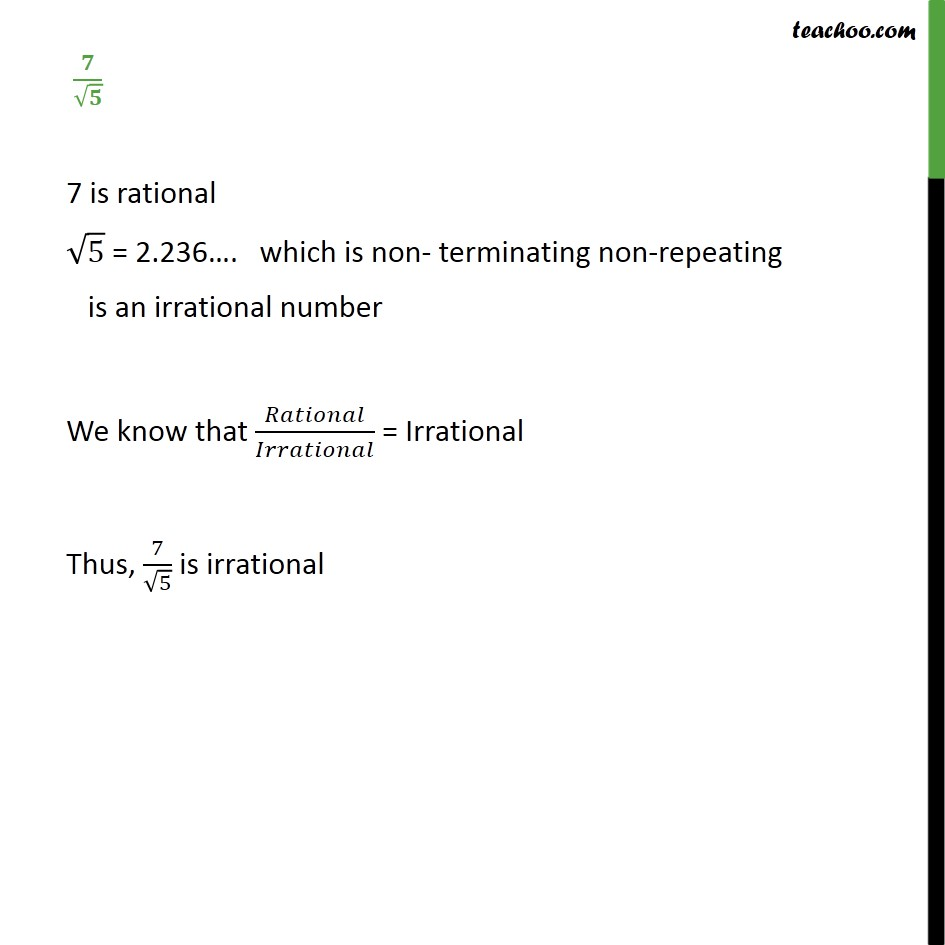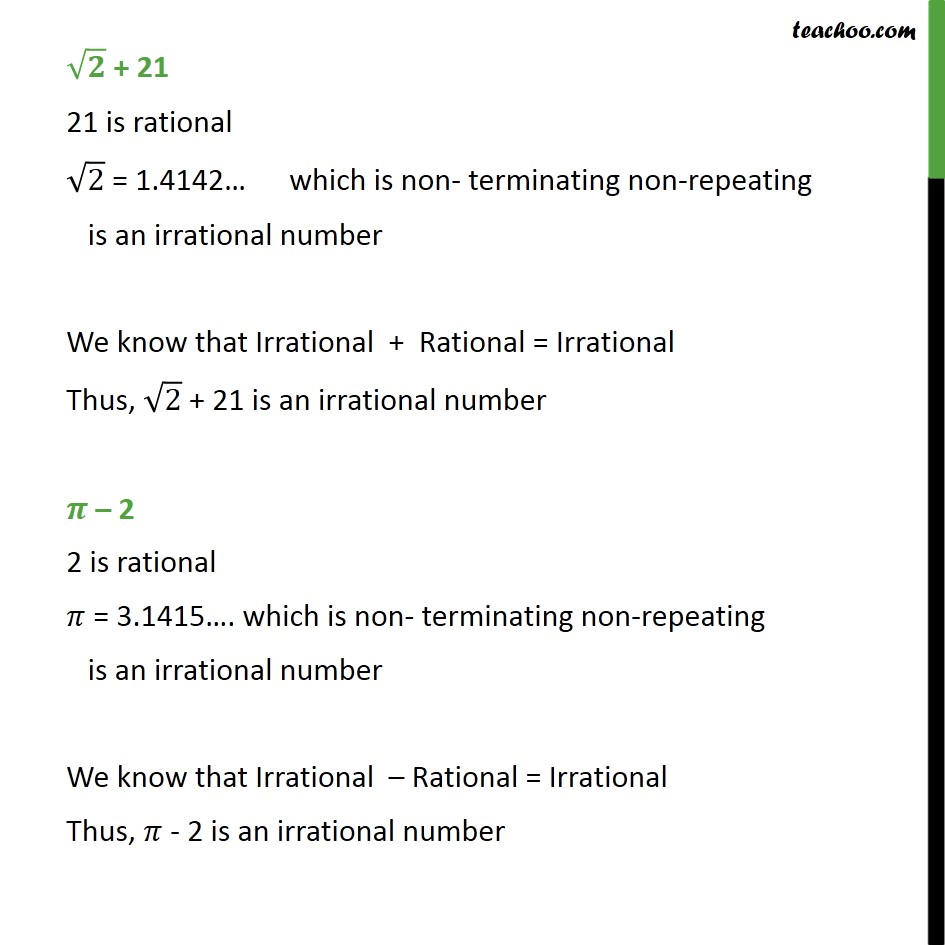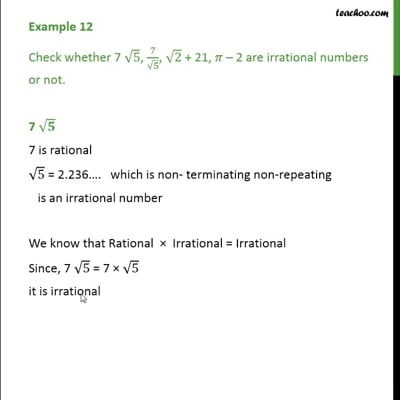This video is only available for Teachoo black users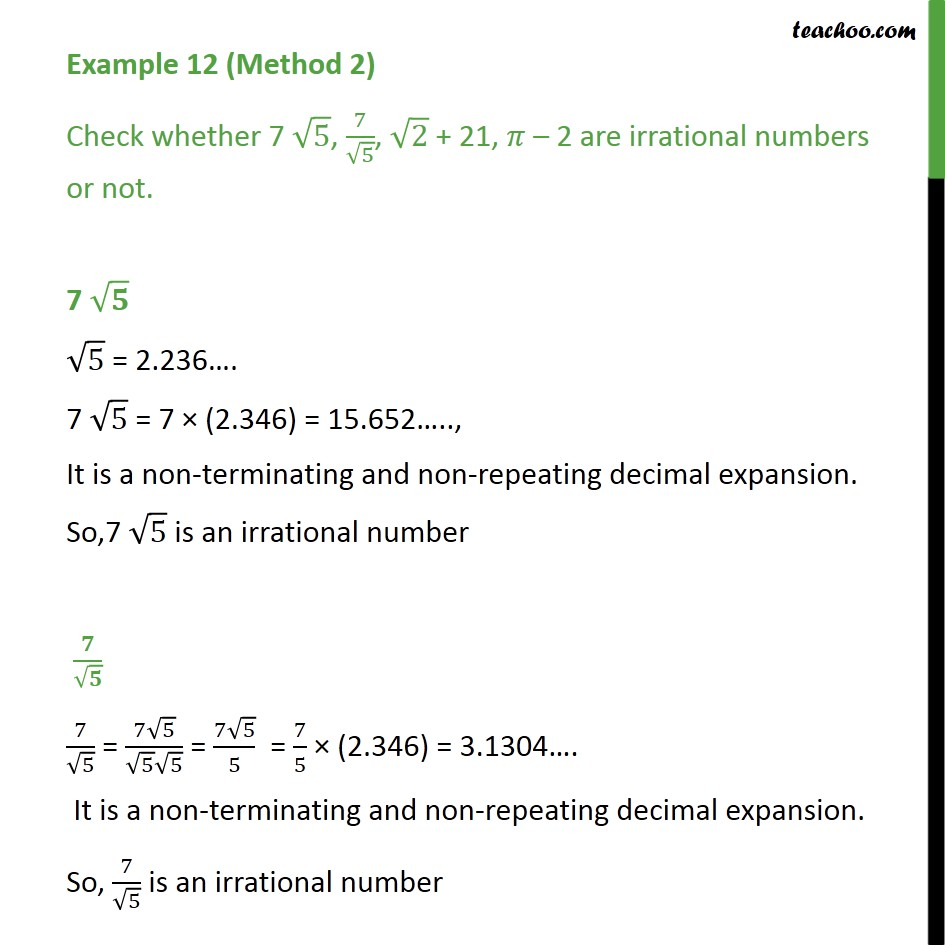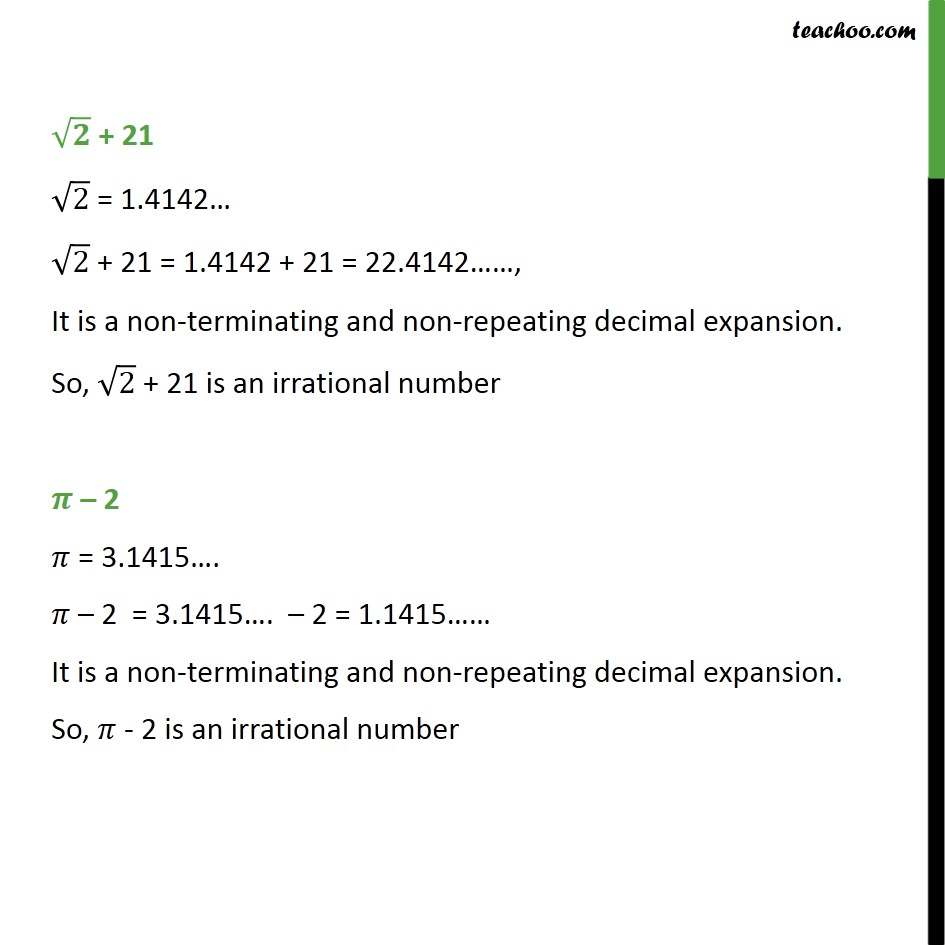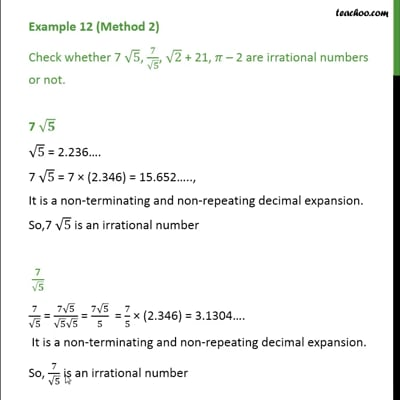This video is only available for Teachoo black users

Learn in your speed, with individual attention - Teachoo Maths 1-on-1 Class

### Transcript

Example 11(Method 1) Check whether 7 5, 7/ 5, 2 + 21, 2 are irrational numbers or not. 7 7 is rational 5 = 2.236 . which is non- terminating non-repeating is an irrational number We know that Rational Irrational = Irrational Since, 7 5 = 7 5 it is irrational / 7 is rational 5 = 2.236 . which is non- terminating non-repeating is an irrational number We know that / = Irrational Thus, 7/ 5 is irrational + 21 21 is rational 2 " = 1.4142 " which is non- terminating non-repeating is an irrational number We know that Irrational + Rational = Irrational Thus, 2 + 21 is an irrational number 2 2 is rational = 3.1415 . which is non- terminating non-repeating is an irrational number We know that Irrational Rational = Irrational Thus, - 2 is an irrational number Example 11 (Method 2) Check whether 7 5, 7/ 5, 2 + 21, 2 are irrational numbers or not. 7 5 = 2.236 . 7 5 = 7 (2.346) = 15.652 .., It is a non-terminating and non-repeating decimal expansion. So,7 5 is an irrational number / 7/ 5 = (7 5)/( 5 5) = (7 5)/5 = 7/5 (2.346) = 3.1304 . It is a non-terminating and non-repeating decimal expansion. So, 7/ 5 is an irrational number + 21 2 = 1.4142 2 + 21 = 1.4142 + 21 = 22.4142 , It is a non-terminating and non-repeating decimal expansion. So, 2 + 21 is an irrational number 2 = 3.1415 . 2 = 3.1415 . 2 = 1.1415 It is a non-terminating and non-repeating decimal expansion. So, - 2 is an irrational number